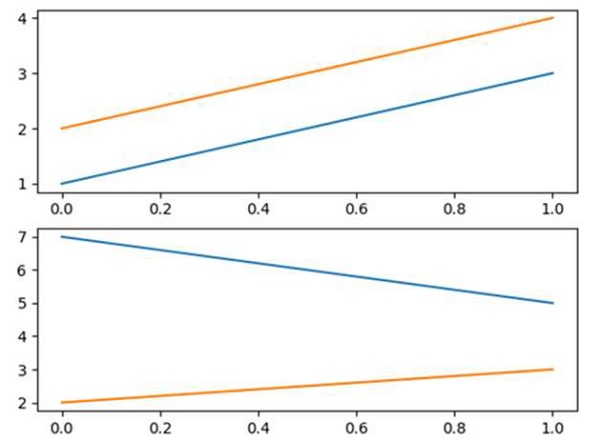# How to make several plots on a single page using Matplotlib?

We can create a figure using plt.figure() method and can create axes using fig.add_subplot(211) where nrow=2 and ncols=1. After that, we can plot the lines over both the plots in a figure.

## Steps

• Using gcf() method, Get the current figure. If no current figure exists, a new one is created using ~.pyplot.figure().

• Add an ~.axes.Axes to the figure as part of a subplot arrangement, using add_subplot() method, where nrows = 2, ncols = 1 and position = 1.

• Plot the line using plt.plot() method at position 1.

• Add an ~.axes.Axes to the figure as part of a subplot arrangement, using add_subplot() method, where nrows = 2, ncols = 1 and position = 2.

• Plot the line using plt.plot() method at position 2.

• To show the figure use plt.show() method.

## Example

from matplotlib import pyplot as plt

fig = plt.figure()

plt.show()